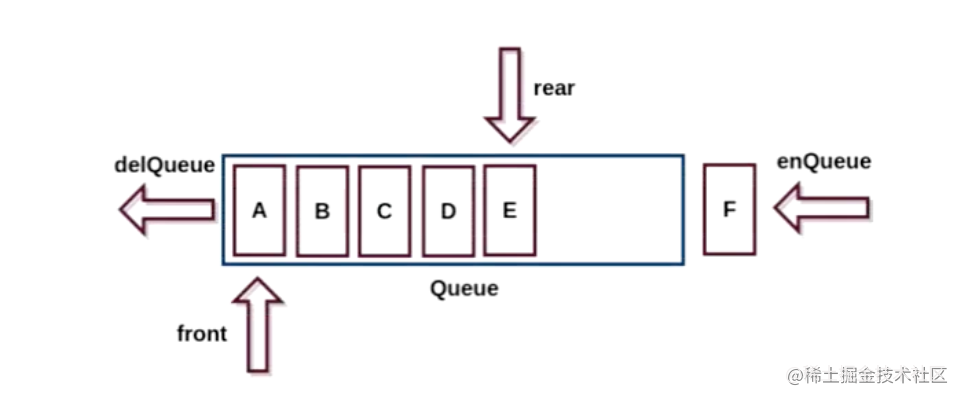2023-02-0200

## 目录

1. 认识队列结构
2. 实现队列结构封装
3. 实战一：最近的请求次数
3.1 题目描述
3.2 解一：队列
4. 实战二：无法吃午餐的学生数量
4.1 题目描述
4.2 解一：队列
5. 实战三：字符串中的第一个唯一字符
5.1 题目描述
5.2 解一：哈希表
5.3 解二：队列

# 1. 认识队列结构

1. 队列是一个 先进先出（FIFO） 的数据结构

2. `js` 中没有队列，但我们可以用 数组或链表 实现队列的所有功能

3. 队列的常用操作:

1. `enqueue(element)`：向队列尾部添加一个（多个）新的项
2. `dequeue()`：移除队列的第一项，并返回被移除的元素
3. `front/peek()`：返回队列中的第一个元素
4. `isEmpty()`：判断队列是否为空
5. `size()`：返回队列的元素个数# 2. 实现队列结构封装

1. 基于 数组 实现
2. 基于 链表 实现

```ts```// 封装一个队列
export default class ArrayQueue<T = any> {
private data: T[] = [];

constructor(data: T[]) {
this.data = data || [];
}

enqueue(element: T): void {
this.data.push(element);
}

dequeue(): T | undefined {
return this.data.shift();
}

peek(): T | undefined {
return this.data;
}

isEmpty(): boolean {
return this.data.length === 0;
}

size(): number {
return this.data.length;
}
}
``````

```ts```const queue = new ArrayQueue<number>();

queue.push(1);
queue.push(2);
queue.pop();
queue.push(3);
console.log(queue); // ArrayQueue { data: [ 2, 3 ] }
``````

# 3. 实战一：最近的请求次数

## 3.1 题目描述

• `RecentCounter()` 初始化计数器，请求数为 0 。
• `int ping(int t)` 在时间 `t` 添加一个新请求，其中 `t` 表示以毫秒为单位的某个时间，并返回过去 `3000` 毫秒内发生的所有请求数（包括新请求）。确切地说，返回在 `[t-3000, t]` 内发生的请求数。

``````输入：
["RecentCounter", "ping", "ping", "ping", "ping"]
[[], , , , ]

[null, 1, 2, 3, 3]

RecentCounter recentCounter = new RecentCounter();
recentCounter.ping(1);     // requests = ，范围是 [-2999,1]，返回 1
recentCounter.ping(100);   // requests = [1, 100]，范围是 [-2900,100]，返回 2
recentCounter.ping(3001);  // requests = [1, 100, 3001]，范围是 [1,3001]，返回 3
recentCounter.ping(3002);  // requests = [1, 100, 3001, 3002]，范围是 [2,3002]，返回 3
``````

• `1 <= t <= 109`
• 保证每次对 `ping` 调用所使用的 `t` 值都 严格递增
• 至多调用 `ping` 方法 `104` 次

## 3.2 解一：队列

```ts```class RecentCounter {
queue: ArrayQueue<number>;

constructor() {
this.queue = new ArrayQueue<number>();
}

ping(t: number): number {
this.queue.enqueue(t);
while (this.queue.peek() < t - 3000) {
this.queue.dequeue();
}
return this.queue.size();
}
}

/**
* Your RecentCounter object will be instantiated and called as such:
* var obj = new RecentCounter()
* var param_1 = obj.ping(t)
*/
``````

• 时间复杂度：均摊 `O(1)`，每个元素至多入队出队各一次。

• 空间复杂度：`O(L)`，其中 `L` 为队列的最大元素个数。

# 4. 实战二：无法吃午餐的学生数量

## 4.1 题目描述

• 如果队列最前面的学生 喜欢 栈顶的三明治，那么会 拿走它 并离开队列。
• 否则，这名学生会 放弃这个三明治 并回到队列的尾部。

``````输入： students = [1,1,0,0], sandwiches = [0,1,0,1]

- 最前面的学生放弃最顶上的三明治，并回到队列的末尾，学生队列变为 students = [1,0,0,1]。
- 最前面的学生放弃最顶上的三明治，并回到队列的末尾，学生队列变为 students = [0,0,1,1]。
- 最前面的学生拿走最顶上的三明治，剩余学生队列为 students = [0,1,1]，三明治栈为 sandwiches = [1,0,1]。
- 最前面的学生放弃最顶上的三明治，并回到队列的末尾，学生队列变为 students = [1,1,0]。
- 最前面的学生拿走最顶上的三明治，剩余学生队列为 students = [1,0]，三明治栈为 sandwiches = [0,1]。
- 最前面的学生放弃最顶上的三明治，并回到队列的末尾，学生队列变为 students = [0,1]。
- 最前面的学生拿走最顶上的三明治，剩余学生队列为 students = ，三明治栈为 sandwiches = 。
- 最前面的学生拿走最顶上的三明治，剩余学生队列为 students = []，三明治栈为 sandwiches = []。

``````

``````输入： students = [1,1,1,0,0,1], sandwiches = [1,0,0,0,1,1]

``````

• `1 <= students.length, sandwiches.length <= 100`
• `students.length == sandwiches.length`
• `sandwiches[i]` 要么是 `0` ，要么是 `1` 。
• `students[i]` 要么是 `0` ，要么是 `1` 。

## 4.2 解一：队列

```ts```function countStudents(students: number[], sandwiches: number[]): number {
const studentsQueue = new ArrayQueue<number>(students);
const sandwichesQueue = new ArrayQueue<number>(sandwiches);

let count = 0;
while (studentsQueue.size()) {
if (studentsQueue.peek() === sandwichesQueue.peek()) {
studentsQueue.dequeue();
sandwichesQueue.dequeue();
count = 0;
} else {
studentsQueue.enqueue(studentsQueue.dequeue()!);
count++;
}

if (count === studentsQueue.size()) return sandwichesQueue.size();
}

return 0;
}
``````

• 时间复杂度：`O(n)`，其中 `n` 是学生的数量。
• 空间复杂度：`O(1)`

# 5. 实战三：字符串中的第一个唯一字符

## 5.1 题目描述

``````输入: s = "leetcode"

``````

``````输入: s = "loveleetcode"

``````

``````输入: s = "aabb"

``````

• `1 <= s.length <= 105`
• `s` 只包含小写字母

## 5.2 解一：哈希表

1. 第一次遍历存储每个字符对应的出现次数
2. 第二次遍历取出第一个只出现第一次的字符

```ts```function firstUniqChar(s: string): number {
const map = new Map<string, number>();
for (let i = 0; i < s.length; i++) {
let n = map.get(s[i]);
n ? map.set(s[i], n + 1) : map.set(s[i], 1);
}

for (let i = 0; i < s.length; i++) {
if (map.get(s[i]) === 1) return i;
}
return -1;
}
``````

• 时间复杂度：`O(n)`
• 空间复杂度：`O(∣Σ∣)`，其中 `Σ` 是字符集，在本题中 `s` 只包含小写字母，因此 `∣Σ∣≤26` 。我们需要 `O(∣Σ∣)` 的空间存储哈希映射。

## 5.3 解二：队列

```ts```function firstUniqChar(s: string): number {
const map = new Map<string, number>();
const queue = new ArrayQueue<[string, number]>();
for (let i = 0; i < s.length; i++) {
if(!map.has(s[i])) {
map.set(s[i], i)
queue.enqueue([s[i], i]);
} else {
map.set(s[i], -1)
while(queue.size() && map.get((queue.peek() as [string,number])) === -1) {
queue.dequeue()
}
}
}

return queue.size() ? (queue.peek() as [string, number]) : -1;
}
``````

• 时间复杂度：`O(n)`
• 空间复杂度：`O(∣Σ∣)`